# Calculated Field

Erstellt von Igor Galic, Geändert am Wed, 03 Aug 2022 um 12:55 PM von Igor Galic

First thing that is important is that calculated field can be only used with "number" value fields. Because SyncSpider has to register the calculation with numbers, as we will be adding VAT Price in % for the example that we will be using.

Lets say we are trying to transfer the price from Dokan to Shopify. As Shopify is expecting the price to be with VAT, but Dokan only has "Price" ( meaning without VAT) in this case we use calculated field.

So we go to the Configuration of Value Types for Dokan, and change the value type from "text" to "Number"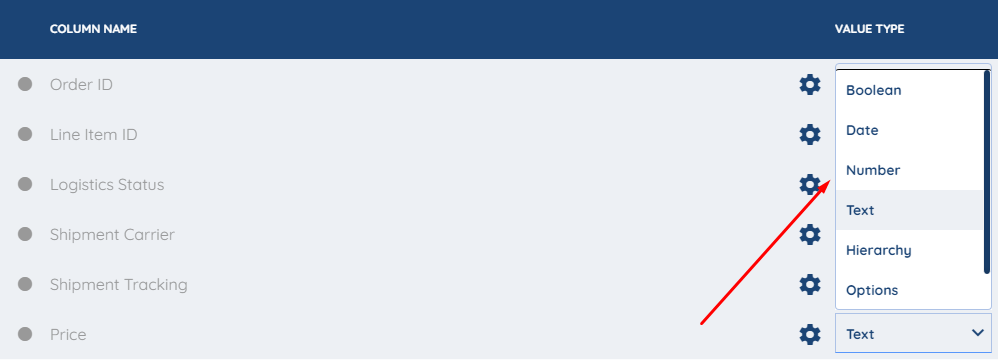We can leave the settings for the "Price" field as they are, for this usecase.Now we move to the mapping part, Here is where you create the "Calculated" Field.

On the bottom left corner on mapping click on the + sign and click on "Calculated Field".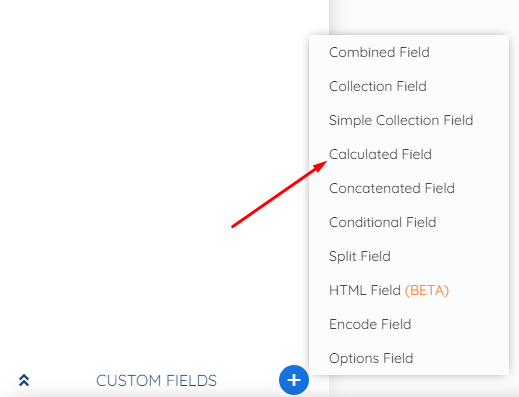Here are the options for Calculated field that we will be explaining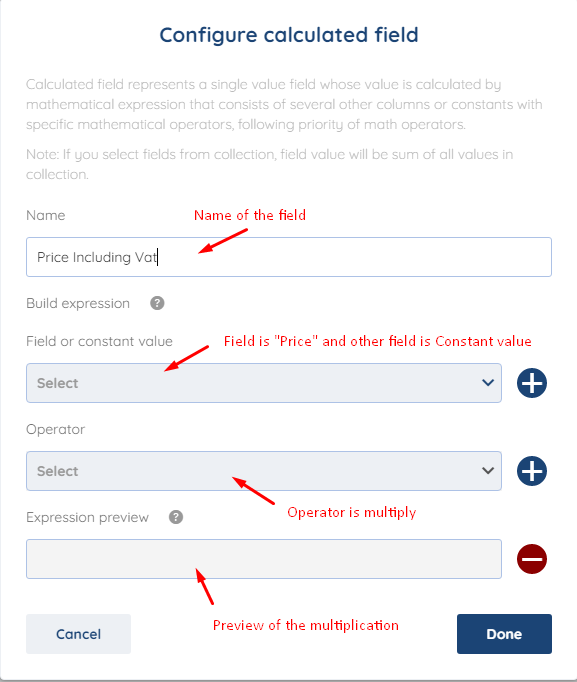First you will name the field. You can put "Price Including Vat" or whatever you prefer but we would recommend that you put the % of VAT in the name of the field.

Next we add the "Price" Field that we were changing earlier and click on the + to add it to the Expression preview.Now we will add the Operator "*" which in this case is "Multiply" as we are adding 20% ( this varies from usecase to usecase)  to the Price. When adding the VAT you Multiply the original number with the % of the VAT, so in this case it will be Price * 1.2 ( as 20% is 0.2 of the original Price)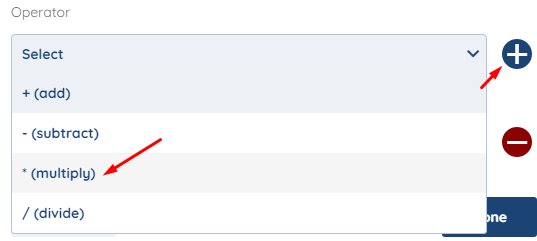To add the % of VAT, now we add another field which is constant Value and set it to 1.2 as explained above how the multiplication of VAT works.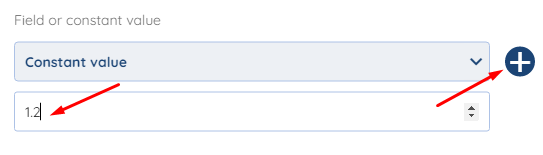When we have added all the fields and Operator for this example, the preview should look something like this.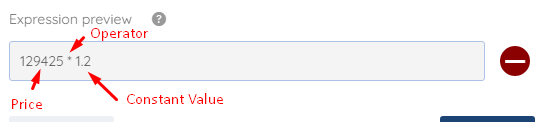When you have created it, just pull it from the bottom left corner and connect it to the "Price" of Shopify in the mapping section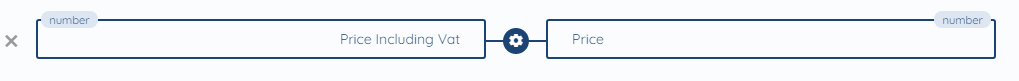This is all for the Calculated Field,